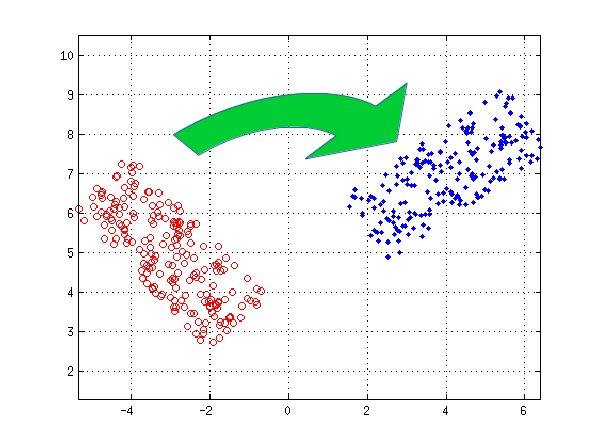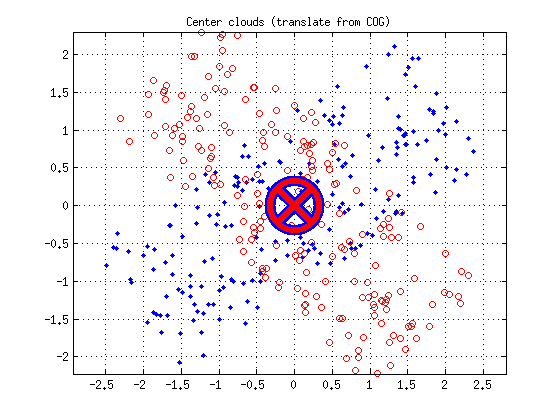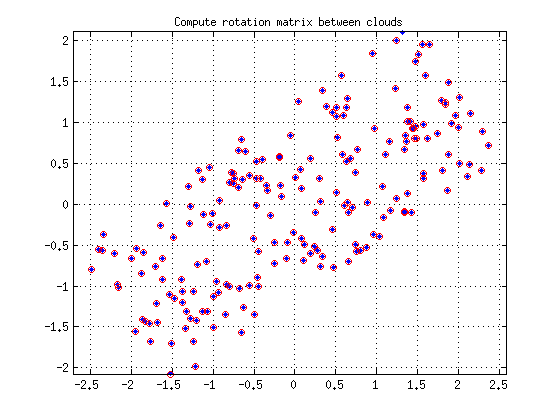Do me a favor, take a few seconds to have a look at my last project.

Thank you, Lulu

# Calculating the transformation between two set of points

## Hypotheses

The aim of this article is to minimize the error between two clouds of points (minimization of the point to point error). Let’s considere the following cloud of points A in blue and B in red. Our goal is to find the transformation of B (translation and rotation) in order to minimize the distance point to point (according to the least square minimization).$$A =\left[ \begin{matrix} p_1^a & p_2^a & ... & p_n^a \end{matrix} \right] =\left[ \begin{matrix} x_1^a & x_2^a & ... & x_n^a \ y_1^a & y_2^a & ... & y_n^a \end{matrix} \right]$$

$$! B =\left[ \begin{matrix} p_1^b & p_2^b & ... & p_n^b \end{matrix} \right] =\left[ \begin{matrix} x_1^b & x_2^b & ... & x_n^b \ y_1^b & y_2^b & ... & y_n^b \end{matrix} \right]$$

We assume that the point matching has already being performed: each point of $$A$$ is associated with a point of $$B$$.## Translation

First, compute the center of gravity of each cloud:$$\begin{matrix}COG^a=\frac{1}{n}. \sum\limits_{i=1}^n p_i^a & COG^b=\frac{1}{n}. \sum\limits_{i=1}^n p_i^b \end{matrix}$$

Translate each point with a translation vector given by the center of gravity of its cloud (center each cloud on the origin).

$$\begin{matrix} A'=A-COG^a & B'=B-COG^b \end{matrix}$$## Rotation

The rotation is a little bit more tricky. First calculate the following matrix:

$$N = \sum\limits_{i=1}^n {p'}_i^b.{{p'}_i^a}^T = \sum\limits_{i=1}^n \left[ \begin{matrix} {x'}_i^b \ {y'}_i^b \end{matrix} \right]. \left[ \begin{matrix} {x'}_i^a & {y'}_i^a \end{matrix} \right]$$

Compute the singular value decomposition of $$N$$:

$$N=U.\Sigma.V^T$$

The rotation matrix is given by:

$$R=V.U^T$$

The rotation angle can now be extracted from the matrix $$R$$:

$$\alpha=atan2(R_{21},R_{11})$$

By applying the rotation on the previously translated set of points, we get the following result:## Download

Matlab source code (example on this page) can be download here:

cloudTrans_matlab.zip

Credits: based on the very good bachelor thesis of Hans Martin Kjer and Jakob Wilm: Evaluation of surface registration algorithms for PET motion correction.

## See also

Last update : 04/13/2019